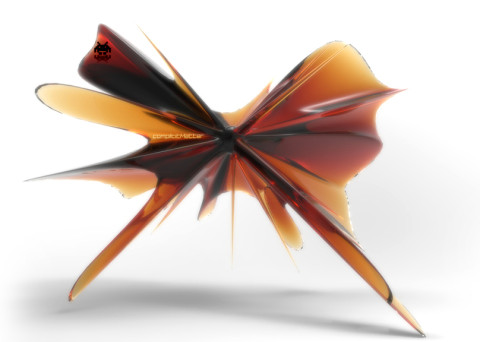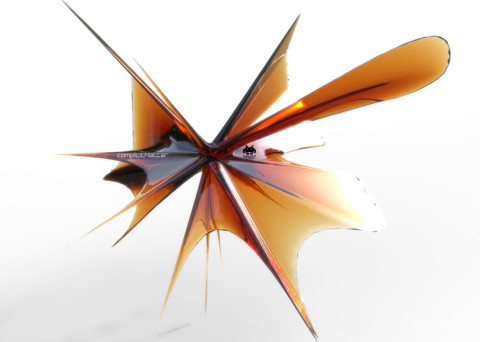# [C]reepers

### Gallery details

Code Creates Random Creeper Agents which deform a mesh. Mesh Vertex Colors are based on Deformation Values compared to the rest state – C# By Luis Quinones & Andrew Reitz

—Quick Study on deforming meshes based on random  creeper agents in space. Similar to [BALLED] study but here instead of using nDynamics and keyframes in Maya to deform the mesh we will use agents in Grasshopper. The iterations are controlled by the GH Timer . Each creeper agent has an amount of closest points they can influence and that influence can either be controlled by an amplitude vector or by the distance to the  creeper agent position. This study also implementsto create the  creeper agent geometry—
••••••••Using Grasshopper C# components for the code snippets below  – All Test code is provided on an ‘as-is’ basis for evaluation, testing and commenting purposes. I am not responsible for any damage incurred by its usage. Use at your own risk.

### Base Creeper Agent Class - C# Code

```//Code written by Luis Quinones @ [complicitMatter]
//Feel free to use and modify the code in any way you want, please comment below with suggestions, ideas of any relevant throughts

private void RunScript(Point3d att, bool reset, int ptCount, ref object A)
{
BoundingBox bb = new BoundingBox(-100, -100, -100, 100, 100, 100); //set bounding box
Random rnd = new Random();

List<Vector3d> vecs = new List<Vector3d>();
List<Point3d> returnedvecs = new List<Point3d>();

if(reset){ //reset will re initialize all values, since we are using the timer we will use this as a trigger

this.startList = new List<Vector3d>();

for(int i = 0; i < ptCount; i++){
this.startPos = new Vector3d(rnd.Next(-100, 100), rnd.Next(-100, 100), rnd.Next(-100, 100)); //set the starting position

this.moveVec = new Vector3d(rnd.NextDouble(), rnd.NextDouble(), rnd.NextDouble()); //set the move vec
int switchDir = rnd.Next(-1, 1); //use trigger to make some random values negative doubles
if(switchDir < 0){
this.moveVec *= 2;
}else{
this.moveVec *= -2;
}
}
creeperPoints = this.startList;

}else{

creepers creeperPts = new creepers(this.startList, this.moveList, bb); //create instance of creepers
creeperPts.move();

this.startList = creeperPts.outputList;
creeperPoints = this.startList;
}
}
//------------Global Variables--------------------
private List<Vector3d> moveList = new List<Vector3d>();
private List<Vector3d> startList = new List<Vector3d>();
private Vector3d startPos = new Vector3d();
private Vector3d moveVec;
//------------Creeper Class Agents------------------
public class creepers{
//---------------Class Variables--------------------
private BoundingBox bbox;
public Vector3d pos = new Vector3d();
public List<Vector3d> posList = new List<Vector3d>();
public List<Vector3d> posMoveList = new List<Vector3d>();
public List<Vector3d> outputList = new List<Vector3d>();
//-----------------Constructor----------------------
public creepers(List<Vector3d> vecs, List<Vector3d> move, BoundingBox bb){

this.posList = vecs;
this.posMoveList = move;
this.bbox = bb;
}
//-----------------Move method----------------------
public void move(){

for(int i = 0; i < this.posList.Count; i++){

this.pos = new Vector3d();
this.pos += this.posList[i];
this.pos += this.posMoveList[i];

this.checkLoc();

}
}
//------------Check location method----------------
//if the creeper is past the bounding box then spawn in a new location inside
public void checkLoc(){

if(!this.bbox.Contains((Point3d) this.pos)){
Random rnd = new Random();
this.pos = new Vector3d(rnd.Next(-100, 100), rnd.Next(-100, 100), rnd.Next(-100, 100));
}
}
}
Update
```

### Mesh Deform - C# Code

```//Code written by Luis Quinones @ [complicitMatter]
//Feel free to use and modify the code in any way you want, please comment below with suggestions, ideas of any relevant throughts

private void RunScript(Mesh meshIn, List<Point3d> attrPts, int cPCount, double vAmp, bool toggle, ref object C, ref object A)
{
Rhino.Geometry.Collections.MeshVertexList vList = meshIn.Vertices;
Rhino.Geometry.Collections.MeshFaceList fList = meshIn.Faces;

List<Point3f> vertToList = vList.ToList();
List<Point3d> vertPtList = vertToList.ConvertAll(x => (Point3d) x); //convert vertexlist from point3f to point3d
List<Point3d> vertPtDup = new List<Point3d>(vertPtList);

List<Point3d> vertAsPoint = new List<Point3d>();

foreach(Point3d pt in attrPts){ //go through each attractor point (creepers)

for(int i = 0; i < cPCount; i++){ //for however many closestpoints you want each attractor to have

int cIndex_A = Rhino.Collections.Point3dList.ClosestIndexInList(vertPtList, pt);

Vector3d subVec = Vector3d.Subtract(new Vector3d(pt), new Vector3d(vertPtList[cIndex_A]));
subVec.Unitize();

Vector3d mult = new Vector3d();
if(!toggle){
mult = Vector3d.Multiply(subVec, vAmp); //if toggle is false amplify the vector by the user defined value
}else{
double dist = pt.DistanceTo(vertPtList[cIndex_A]);
mult = Vector3d.Multiply(subVec, dist); //if toggle is true then use the distance to the creeper pos
}

int moveIndex = vertPtDup.IndexOf(vertPtList[cIndex_A]); //get the index of the current closest point

vList.SetVertex(moveIndex, Point3d.Add(mult, vertPtList[cIndex_A])); //set the mesh vertex at the specified index to the new location

vertPtList.RemoveAt(cIndex_A); //remove the previous index from the vertex list to find the next closest point
}
}
creeperMesh = meshIn;
ptsOnMesh = vertAsPoint;
}
Update
```

### Mesh Deform - Python GH Code

```#Code written by Luis Quinones @ [complicitMatter]
#Feel free to use and modify the code in any way you want, please comment below with suggestions, ideas of any relevant throughts
import rhinoscriptsyntax as rs
import ghpythonlib.components as gh

def movePoints(mVerts,flattened,myIndexes,attrPts):
otherIndexList = []
staticList = []
#go through and move the points that are not affected by the attactor
for k in range(len(mVerts)):
if k not in flattened:
otherIndexList.append(k)
mOV = gh.Vector2Pt(mVerts[k], mVerts[k], False) 
otherVertsLoc = gh.Move(mVerts[k], mOV)
staticList.append(otherVertsLoc)

movedList = []
#Go through and move the points that are affected by the attactors
for k in range(len(myIndexes)):
for index in myIndexes[k]: #we are going through a list of list of indexes
myVec = gh.Vector2Pt(mVerts[index],attrPts[k], False)  #create the vector to the correct attractor
myLargeVec = gh.Amplitude(myVec, vAmp)
transVerts = gh.Move(mVerts[index], myLargeVec)
movedList.append(transVerts) #append it to the moved list

return staticList, movedList, otherIndexList

def resortData(mVerts, flattened, staticList, movedList, otherIndexList):
count = 0
comboList = []
#resort everything to match the input list orders
for p in range(len(mVerts)):
if p not in flattened:
comboList.insert(p,staticList[otherIndexList.index(count)]) #insert the static point at the correct index in a new list
if p in flattened:
comboList.insert(p,movedList[flattened.index(count)]) #insert the moved point at the correct index in a new list
count += 1

return comboList

#--------------------------------------------------------------
# Deconstruct Input Mesh
mDecon = gh.DeconstructMesh(meshIn)
mVerts = mDecon
mFaces = mDecon

# create a list of list matching each vertex index to its corresponding attractor pt
myIndexes = []
for m in range (len(attrPts)):
cPCalc = gh.ClosestPoints(attrPts[m], mVerts, cPCount)
myCP = cPCalc
myIndexBase = cPCalc
myIndexes.append(myIndexBase)
dupIndexList = myIndexes[:] #dup list
flattened = [val for sublist in dupIndexList for val in sublist] #flatten it

#------------------Build Functions-----------------------------
pointSet = movePoints(mVerts,flattened,myIndexes,attrPts)
newMeshVertices = resortData(mVerts, flattened, pointSet, pointSet, pointSet)
#--------------------------------------------------------------

meshOut = gh.ConstructMesh(newMeshVertices, mFaces) #build that ish
Update
```
• Share